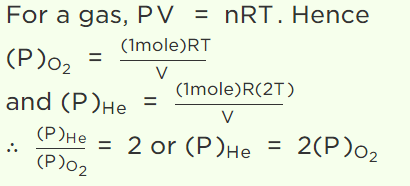## Kinetic Theory Questions and Answers Part-5

1. The pressure P, volume V and temperature T of a gas in the jar A and the other gas in the jar B at pressure 2P, volume V/4 and temperature 2T, then the ratio of the number of molecules in the jar A and B will be
a) 1 : 1
b) 1 : 2
c) 2 : 1
d) 4 : 1

Explanation:2. We write the relation for Boyle's law in the form PV = C when the temperature remains constant. In this relation, the magnitude of C depends upon
a) The nature of the gas used in the experiment
b) The magnitude of g in the laboratory
c) The atmospheric pressure
d) The quantity of the gas enclosed

Explanation: We write the relation for Boyle's law in the form PV = C when the temperature remains constant. In this relation, the magnitude of C depends upon the quantity of the gas enclosed

3. If a given mass of gas occupies a volume of 10 cc at 1 atmospheric pressure and temperature of 100°C(373.15 K). What will be its volume at 4 atmospheric pressure; the temperature being the same
a) 100 cc
b) 400 cc
c) 2.5 cc
d) 104 cc

Explanation:4. A sample of an ideal gas occupies a volume V at a pressure P and absolute temperature T, the mass of each molecule is m. The expression for the density of gas is (k = Boltzmann’s constant)
a) mkT
b) P/kT
c) P/kTV
d) Pm/kT

Explanation: A sample of an ideal gas occupies a volume V at a pressure P and absolute temperature T, the mass of each molecule is m. The expression for the density of gas is Pm/kT

5. The product of the pressure and volume of an ideal gas is
a) A constant
b) Approx. equal to the universal gas constant
c) Directly proportional to its temperature
d) Inversely proportional to its temperature

Explanation: The product of the pressure and volume of an ideal gas is directly proportional to its temperature

6. A balloon contains 500 m3 of helium at 27°C and 1 atmosphere pressure. The volume of the helium at – 3°C temperature and 0.5 atmosphere pressure will be
a) $500 m^{3}$
b) $700 m^{3}$
c) $900 m^{3}$
d) $1000 m^{3}$

Explanation:7.A vessel contains 1 mole of $O_{2}$ gas (molar mass 32) at a temperature T. The pressure of the gas is P. An identical vessel containing one mole of He gas (molar mass 4) at temperature 2T has a pressure of
a) P/8
b) P
c) 2P
d) 8P

Explanation:8. 1 mole of gas occupies a volume of 100 ml at 50 mm pressure. What is the volume occupied by two moles of gas at 100 mm pressure and at same temperature
a) 50 ml
b) 100 ml
c) 200 ml
d) 500 ml

Explanation:9. For ideal gas, which statement is not true
a) It obeys Boyle's law
b) It follows PV = RT
c) Internal energy depends on temperature only
d) It follows Vander-Waal's equation

10. A given mass of a gas is allowed to expand freely until its volume becomes double. If $C_{b}$ and $C_{a}$ are the velocities of sound in this gas before and after expansion respectively, then $C_{a}$ is equal to
a) $2C_{b}$
b) $\sqrt{2}C_{b}$
c) $C_{b}$
d) $\frac{1}{\sqrt{2}}C_{b}$
Explanation: A given mass of a gas is allowed to expand freely until its volume becomes double. If $C_{b}$ and $C_{a}$ are the velocities of sound in this gas before and after expansion respectively, then $C_{a}$ is equal to $C_{b}$